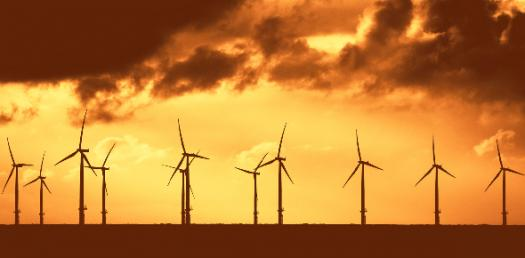# Wind Turbine

5 Questions | Total Attempts: 69SettingsThis quiz is going to be about wind turbines.

• 1.
How old are wind turbines?
• A.

5,000 years old

• B.

2,000 years old

• C.

500 years old

• D.

10,000 years old

• E.

1,000 years old

• 2.
What pushes the electrons in a motor?
• A.

Coils

• B.

• C.

Magnets

• D.

All of Above

• 3.
What can wind turbines do?
• A.

Pump water from wells

• B.

Make electricity

• C.

All of above

• 4.
How many kilowatts can a wind turbine generate?
• A.

1,000 Kilowatts

• B.

800 Kilowatts

• C.

56 Kilowatts

• D.

106 Kilowatts

• E.

600 Kilowatts

• 5.
THow much speed does a wind turbine need to make the blades spin and to create electricity?
• A.

29 Miles

• B.

106 Miles

• C.

10 Miles

• D.

51 Miles

• E.

87 Miles

Related Topics